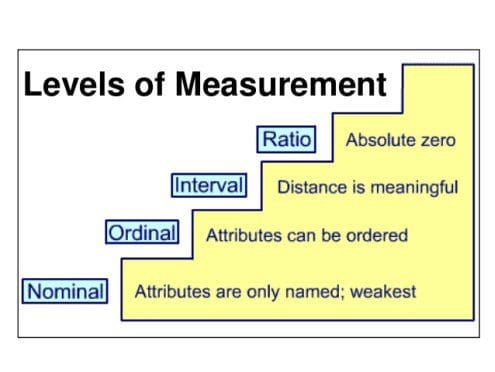# Statistics – Understanding the Levels of Measurement

For doing statistics or analytics it is first step to understand the variables. Moreover, it is important that one truly knows which measure to take with different available types.

By Saurabh Agrawal and Prasad Pande (RideOnData).One of the most important and basic step in learning Statistics is understanding the levels of measurement for the variables. Let’s take a step back and first look at what a variable is? A variable is any quantity that can be measured and whose value varies through the population. For example, if we consider a population of students, the student’s nationality, marks, grades, etc are all the variables defined for the entity student, and their corresponding value will differ for each student. Looking at the larger picture, if we want to compute the average salary of the US citizens, we can go out and record the salary of each and every person to compute the average or choose a random sample from the entire population and compute the average salary for that sample, and then use the statistical tests to derive conclusions for a wider population.

The type of statistical test that can be used to derive a conclusion about the wider population depends upon the level of measurement of the variable under consideration. The level of measurement of a variable is nothing but the mathematical nature of a variable or, how a variable is measured.Broadly, there are 4 levels of measurement for the variables –

1. Nominal Level:

The nominal level variables are organized into non-numeric categories that cannot be ranked or compared quantitatively. So it puts the variables into some categories. These categories of variables has no ordering and are mutually exclusive (i.e each case can only fit into one category) and exhaustive (i.e there is a category for each possible case). Eg: Shoes can be categorized based on type (sports, casual, others) or color (black, brown, others). These categories of shoes has no ordering (greater than, less than, equal to), are mutually exclusive and exhaustive. Hence the type variable for entity shoe is measured at nominal level.

2. Ordinal Level:

In the ordinal level of measurement, the variables are still classified into categories, but these categories are ordered and there is no equivalent distance between the categories. Eg: class variable for a person can have values like upper class, lower class, middle class etc. These values puts a person into a particular category and there is also a defined relative ordering between the classes like upper class > midde class > lower class. But there is no equivalent distance or boundaries between these classes, hence the class variable is measured at the ordinal level of measurement. The categories still must be mutually exclusive and exhaustive, but ​also​ have a logical order that allows them to be ranked.

3. Interval Level:

In the interval level of measurement, the variables are still classified into ordered categories, but there is an equivalent distance between these categories. This allows for a direct comparison between categories such that the difference between any two sequential data points is exactly the same as the difference between any other two sequential data points. The problem with interval level variables is that there is an arbitrary zero point i.e we can only add and subtract two interval level variables but we can’t multiply or divide them. Eg: Shoe size. We can say that the difference between size 3 and size 4 shoe is equal to the distance between size 7 and size 8 shoe, but size 6 shoe is not equal to 2 * size 3 shoe. Also, size 0 shoe does not mean that there is no shoe, its simply a shoe with zero size i.e an arbitrary zero point.

4. Ratio Level:

The ratio level variables have all of the characteristics of nominal, ordinal and interval variables, but also have a meaningful zero point. So the zero point is real and not arbitrary, and a value of zero actually means there is nothing. So we can add, subtract, divide and multiply the two ratio level variables. Eg: Weight of a person. It has a real zero point, i.e zero weight means that the person has no weight. Also, we can add, subtract, multiply and divide weights at the real scale for comparisons.

Each statistical test is designed to be used with variables of the particular level of measurement. So if we can determine a variable’s level of measurement, we can find the statistical tests to be used to reach a conclusion by computing the variable under consideration for a random sample of population. Sometimes a nominal level variable e.g.: race can be misinterpreted as the interval level. Eg: 1 – White, 2 – Black. Simply adding numbers to the nominal level variables doesn’t make them the ordinal or interval level variables.

Related: